4.7.1 Aside: Root Insertion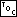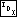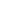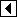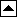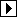One side effect of the usual method for BST insertion, implemented in the previous section, is that items inserted more recently tend to be farther from the root, and therefore it takes longer to find them than items inserted longer ago. If all items are equally likely to be requested in a search, this is unimportant, but this is regrettable for some common usage patterns, where recently inserted items tend to be searched for more often than older items.

In this section, we examine an alternative scheme for insertion that addresses this problem, called “insertion at the root” or “root insertion”. An insertion with this algorithm always places the new node at the root of the tree. Following a series of such insertions, nodes inserted more recently tend to be nearer the root than other nodes.

As a first attempt at implementing this idea, we might try simply making the new node the root and assigning the old root as one of its children. Unfortunately, this and similar approaches will not work because there is no guarantee that nodes in the existing tree have values all less than or all greater than the new node.

An approach that will work is to perform a conventional insertion as a leaf node, then use a series of rotations to move the new node to the root. For example, the diagram below illustrates rotations to move node 4 to the root. A left rotation on 3 changes the first tree into the second, a right rotation on 5 changes the second into the third, and finally a left rotation on 1 moves 4 into the root position:

The general rule follows the pattern above. If we moved down to the left from a node x during the insertion search, we rotate right at x. If we moved down to the right, we rotate left.

The implementation is straightforward. As we search for the insertion point we keep track of the nodes we've passed through, then after the insertion we return to each of them in reverse order and perform a rotation:

```33. <BST item insertion function, root insertion version 33> =
void **bst_probe (struct bst_table *tree, void *item) {
<rb_probe() local variables; rb => bst 198>

<Step 1: Search BST for insertion point, root insertion version 34>
<Step 2: Insert new BST node, root insertion version 35>
<Step 3: Move BST node to root 36>

return &n->bst_data;
}
```

```34. <Step 1: Search BST for insertion point, root insertion version 34> =
pa = (struct bst_node *) &tree->bst_root;
da = 0;
k = 1;
for (p = tree->bst_root; p != NULL; p = p->bst_link[da[k - 1]])   {
int cmp = tree->bst_compare (item, p->bst_data, tree->bst_param);
if (cmp == 0)
return &p->bst_data;

if (k >= BST_MAX_HEIGHT)       {
bst_balance (tree);
return bst_probe (tree, item);
}

pa[k] = p;
da[k++] = cmp > 0;
}
```

This code is included in 33.

```35. <Step 2: Insert new BST node, root insertion version 35> =
n = pa[k - 1]->bst_link[da[k - 1]] =
tree->bst_alloc->libavl_malloc (tree->bst_alloc, sizeof *n);
if (n == NULL)
return NULL;

n->bst_data = item;
tree->bst_count++;
tree->bst_generation++;
```

This code is included in 33.

```36. <Step 3: Move BST node to root 36> =
for (; k > 1; k–)   {
struct bst_node *q = pa[k - 1];

if (da[k - 1] == 0)       {
}     else /* da[k - 1] == 1 */       {
}
pa[k - 2]->bst_link[da[k - 2]] = n;
}
```

This code is included in 33, 622, and 627.

Exercises:

1. Root insertion will prove useful later when we write a function to join a pair of disjoint BSTs (see Joining BSTs). For that purpose, we need to be able to insert a preallocated node as the root of an arbitrary tree that may be a subtree of some other tree. Write a function to do this matching the following prototype:

```static int root_insert (struct bst_table *tree, struct bst_node **root,
struct bst_node *new_node);
```

Your function should insert new_node at *root using root insertion, storing new_node into *root, and return nonzero only if successful. The subtree at *root is in tree. You may assume that no node matching new_node exists within subtree root. [answer]

2. Now implement a root insertion as in Exercise 1, except that the function is not allowed to fail, and rebalancing the tree is not acceptable either. Use the same prototype with the return type changed to void. [answer]

*3. Suppose that we perform a series of root insertions in an initially empty BST. What kinds of insertion orders require a large amount of stack? [answer]